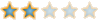Montreal Math ClubMathematics students in Montreal - Étudiants de mathématiques à MontréalHomeCalendarFAQSearchMemberlistUsergroupsRegisterLog in

 Montreal Math Club :: Mathematics :: Combinatorics Share

Combinatorial FieldsAuthorMessage

EuclidPosts : 95
Join date : 2009-09-15
Age : 57
Location : AlexandriaSubject: Combinatorial FieldsThu Aug 25, 2011 12:04 pm hi, here is another good news,I just found in the Carmichael book 1937 http://books.google.ca/books/about/Introduction_to_the_theory_of_groups_of.html?id=sd5QAAAAMAAJchapter XIII is dedicated to the one-to-one correspondence between sharply double transitive groups and near-fields.hence, we have another algebraical structure to be described in combinatorial terms:aNear-field' = aGrouprecall that aField' = aCyc (defining the combinatorial fields) take the tetrahedron as example : there are 12 rotations that permute 4 verticesTetrahedron' = Cycafter fixing a vertex with the finger, the remaining liberty of rotation (relabeling) is a cyc here (page 7) is shown how to introduce coordinates (is a very fast job) http://books.google.com/books?id=7DdJaZcJ0bIC&printsec=frontcover&dq=near+rings&hl=en&ei=U9BXToPdK6nb0QHYyZjDDA&sa=X&oi=book_result&ct=result&resnum=2&ved=0CDQQ6AEwAQ#v=onepage&q&f=false- choose a 0- choose a 1since the structure there is a stick-two-stuck-all structure (a combinatorial field), every point got now an identity, a unique determination. Let' take another example, a triangular prism with 6 vertices and 6 rotation,If we choose a vertex (combinatorial stuff) and we name it - let's say A, the other vertices became- the left side of A one- the right side of A one- the correspondent of A in the other triangle- the correspondent of the left side of A one- the correspondent of the right side of A one We have a name-one-all-named process.my conclusion isIf someone wants to do what teacher Descartes asked, i.e. to introduce coordinates, he will need stick-one-stuck-all structures, and stick-two-stuck-all structures,i. e. the primitives of Lin species.After pinning a 0 (and a 1), all the other points get an identity, a unique determination.Being well determined, one can carry now further algebraical operations. In fact, the primitives of Lin, (stick-one-stick-all) structures are studied for 150 years now, and they are known today as groups and fields.In the finite area, the record of sharp-transitivity are detained by the Mathieu groups M11 - the only stick-four-stuck-all groupM12 - the only stick-five-stuck-all group­EuclidPosts : 95
Join date : 2009-09-15
Age : 57
Location : AlexandriaSubject: Re: Combinatorial FieldsWed Aug 31, 2011 3:03 am Fano_plane'' = X.Klein_four-groupAfter pinning any two points in the Fano plane, a third one is determined.The remaining geometry is described by the Klein group acting on itself (also, the P4bic species).======================here is another remark, that could be useful to explain what a combinatorial field (and, thus, a field) is :the so called Complex Plane is not a plane, but is the complex line.Yes, that surface on the blackboard, containing a C letter, a circle, a 0, a 1 and two axes is a LINE, not a PLANE.EuclidPosts : 95
Join date : 2009-09-15
Age : 57
Location : AlexandriaSubject: Re: Combinatorial FieldsThu Sep 08, 2011 11:40 am (modified - I have opened a new topic on projectivity)­Last edited by nick on Tue Sep 20, 2011 5:45 am; edited 1 time in totalEuclidPosts : 95
Join date : 2009-09-15
Age : 57
Location : AlexandriaSubject: Re: Combinatorial FieldsThu Sep 15, 2011 9:35 pm let me mention some other ones,the stick-3-stuck-all structures are already known, they are the projective lines : http://en.wikipedia.org/wiki/Projective_linebut a nice surprise is VectorSpace[n^k]' = Cyc[n-1](Lin [k]) = Cylinderwhere k(n-1) + 1 = n^k,yes, the container for vector spaces is a primitive of a cylinder,given a vector (affine) space V over a field K, one may built the transformations x ----> ax+b wherea is a coefficient in Kb is a vector in Vthis is an imprimitive permutation group, with transitivity higher than 1 and lower than 2.A vector (affine) space is the group #5 of degree 9 in the Conway (Maple) table; the blocks in the table are representatives of lines parallelism classes.If we pin a point in a normal R^2 space, all the translations are killed. However, it remains the overall scaling with some lambda (warning, not rotations, a vector space with rotations is something else) ==============here is a nice notation for a cyc(lin) :start from a permutation - let's say (1 2 3)(4 5 6)(7 8 9)(0 A B) and optimize like this :(1470 258A 369B) <------- this is a cyc3(lin4), the derivative of 9T5 is something like (1234 4678).there are four lines 14, 26, 37, 48 passing through 0, and after pinning the 0 one can synchronously permute the 8 symbolsSubject: Re: Combinatorial FieldsCombinatorial FieldsPage 1 of 1
 Similar topics» DID interview with Therapist Ellie Fields

Permissions in this forum:You cannot reply to topics in this forumJump to: Select a forum||--Math Club|   |--Mathematical Sundays|   |--Suggestions|   |--Introduce yourself|   |--Mathematics|   |--Problem Solving|   |   |--Solved problems|   |   |   |--Calculus and Analysis|   |--Combinatorics|   |--Geometry|   |--Number Theory|   |--Probabilities and statistics|   |--Set theory and Topology|   |--Online Resources|   |--Chit chat    |--The Water Cooler    |--Mathematical poems